Next: Comments on Hilbert's Program Up: The Natural Numbers Previous: Peano's Axioms

## Some Theorems Derivable from Peano's Axioms

The following is quoted from Edmund Landau, Foundations of Analysis, Chelsea, 1951, pp. 1-18.

Theorem 1:
If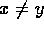then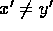.

Theorem 2: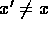.

Theorem 3:
If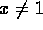there exists one (and hence, by Axiom 4, exactly one) u such that x=u'.

Theorem 4,
and at the same time Definition 1: To every pair of numbers x,y, we may assign in exactly one way a natural number, called x+y (+ to be read ``plus''), such that
1.
x+1=x' for every x.
2.
x+y'=(x+y)' for every x and every y.

x+y is called the sum of x and y, or the number obtained by addition of y to x.

Theorem 5 (Associative Law of Addition):
(x+y)+z=x+(y+z).

Theorem 6 (Commutative Law of Addition):
x+y=y+x.

Theorem 7: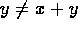.

Theorem 8:
If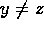then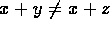.

Theorem 9:
For given x and y, exactly one of the following must be the case:
1.
x=y.
2.
There exists a u (exactly one, by Theorem 8) such that x=y+u.
3.
There exists a v (exactly one, by Theorem 8) such that y=x+v.

Definition 2:
If x=y+u then x>y. (> to be read ``is greater than.'')

Definition 3:
If y=x+v then x<y. (< to be read ``is less than.'')

Theorem 10:
For any given x,y, we have exactly one of the cases x=y, x>y, x<y.

Theorem 11:
If x>y then y<x.

Theorem 12:
If x<y then y>x.

Definition 4: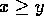means x>y or x=y. (to be read ``is greater than or equal to.'')

Definition 5: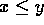means x<y or x=y. (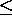to be read ``is less than or equal to.'')

Theorem 13:
Ifthen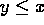.

Theorem 14:
Ifthen.

Theorem 15 (Transitivity of Ordering:)
If x<y, y<z, then x<z.

Theorem 16:
If, y<z or x<y,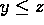, then x<z.

Theorem 17:
If,, then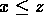.

Theorem 18:
x+y>x.

Theorem 19:
If x>y, or x=y, or x<y, then x+z>y+z, or x+z=y+z, or x+z<y+z, respectively.

Theorem 20:
If x+z>y+z, or x+z=y+z, or x+z<y+z, then x>y, or x=y, or x<y, respectively.

Theorem 21:
If x>y, z>u, then x+z>y+u.

Theorem 22:
If, z>u or x>y,, then x+z>y+u.

Theorem 23:
If,, then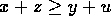.

Theorem 24: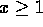.

Theorem 25:
If y>x then.

Theorem 26:
If y<x+1 then.

Theorem 27:
In every non-empty set of natural numbers there is a least one (i.e., one which is less than any other number of the set).

Theorem 28
and at the same time Definition 6: To every pair of numbers x,y, we may assign in exactly one way a natural number, called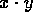(to be read ``times''; however, the dot is usually omitted), such that
1.for every x,
2.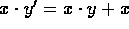for every x and every y.is called the product of x and y, or the number obtained from multiplication of x by y.

Theorem 29 (Commutative Law of Multiplication):
xy=yx.

Theorem 30 (Distributive Law):
x(y+z)=xy+xz.

Theorem 31 (Associative Law of Multiplication):
(xy)z=x(yz).

Theorem 32:
If x>y, or x=y, or x<y, then xz>yz, xz=yz, or xz<yz, respectively.

Theorem 33:
If xz>yz, or xz=yz, or xz<yz, then x>y, or x=y, or x<y, respectively.

Theorem 34:
If x>y, z>u, then xz>yu.

Theorem 35:
If, z>u or x>y,, then xz>yu.

Theorem 36:
If,, then.Next: Comments on Hilbert's Program Up: The Natural Numbers Previous: Peano's Axioms

Carl Lee
Wed Sep 16 09:26:16 EDT 1998